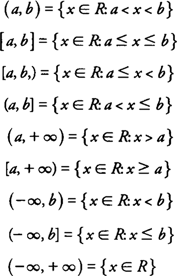## Interval Notation

The set of real numbers ( R) is the one that you will be most generally concerned with as you study calculus. This set is defined as the union of the set of rational numbers with the set of irrational numbers. Interval notation provides a convenient abbreviated notation for expressing intervals of real numbers without using inequality symbols or set‐builder notation.

The following lists some common intervals of real numbers and their equivalent expressions, using set‐builder notation:Note that an infinite end point (±∞) is never expressed with a bracket in interval notation because neither +∞ nor −∞ represents a real number value.